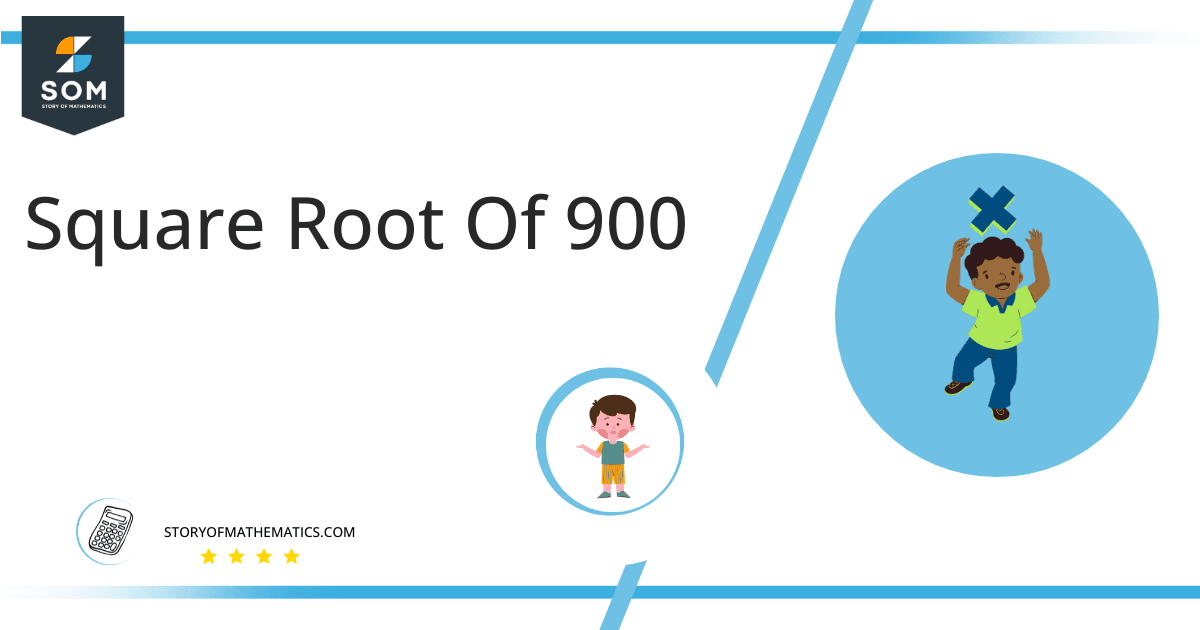# Square Root of 900 + Solution With Free StepsWe can evaluate the square root of a three-digit number using a simple method of long division which is explained in detail below. In this solution, we see the square root of 900 as √900 which is equal to 30 as it has no decimal places so it means 900 is a perfect square and rational as well.

In this article, we will analyze and find the square root of 900 using various mathematical techniques, such as the approximation method and the long division method.

## What Is the Square Root Of 900?

The square root of the number 900 is 30.

The square root can be defined as the quantity that can be doubled to produce the square of that similar quantity. In simple words, it can be explained as:

√900 = √(30 x 30)

√900 = √(30)$^2$

√900 = ±30

The square can be canceled with the square root as it is equivalent to 1/2; therefore, obtaining 30. Hence 30 is 900’s square root. The square root generates both positive and negative integers.

## How To Calculate the Square Root of 900?

You can calculate the square root of 900 using techniques in mathematics that as the Long Division method.

The symbol √ is interpreted as 900 raised to the power 1/2. So any number, when multiplied by itself, produces its square, and when the square root of any squared number is taken, it produces the actual number.

Let us discuss each of them to understand the concepts better.

### Square Root by Long Division Method

The process of long division is one of the most common methods used to find the square roots of a given number. It is easy to comprehend and provides more reliable and accurate answers. The long division method reduces a multi-digit number to its equal parts.

Learning how to find the square root of a number is easy with the long division method. All you need are five primary operations- divide, multiply, subtract, bring down or raise, then repeat.

Following are the simple steps that must be followed to find the square root of 900 using the long division method:

### Step 1

First, write the given number 900 in the division symbol, as shown in figure 1.

### Step 2

Starting from the right side of the number, divide the number 900 into pairs such as 00 and 9.

### Step 3

Now divide the digit 9 by a number, giving a number either 9 or less than 9. Therefore, in this case, the remainder is zero, whereas the quotient is 3.

### Step 4

After this, bring down the next pair 00. Now the dividend is 00. To find the next divisor, we need to double our quotient obtained before. Doubling 3 gives 6; hence consider it as the next divisor.

### Step 5

Now pair 6 with another number to make a new divisor that results in $\leq$ 00 when multiplied with the divisor. If the number is not a perfect square, add pair of zeros to the right of the number before starting division.

### Step 6

Adding 6 to the divisor and multiplying 60 with 0 results in 0 $\leq$ 0. The remainder obtained is 0.

### Step 7

The resulting quotient 30 is the square root of 900. Figure 1 given below shows the long division process in detail:figure 1

### Important points

• The number 900 is a perfect square.
• The number 900 is a rational number.
• The number 900 can be split into its prime factorization.

## Is Square Root of 900 a Perfect Square?

The number 900 is a perfect square. A number is a perfect square if it splits into two equal parts or identical whole numbers. If a number is a perfect square, it is also rational.

A number expressed in p/q form is called a rational number. All the natural numbers are rational. A square root of a perfect square is a whole number; therefore, a perfect square is a rational number.

A number that is not a perfect square is irrational as it is a decimal number. As far as 900 is concerned, it is a perfect square. It can be proved as below:

Factorization of 900 results in 30 x 30 which can also be expressed as 30$^2$.

Taking the square root of the above expression gives:

= √(30$^2$)

= (30$^2$)$^{1/2}$

= 30

This shows that 900 is a perfect square and a rational number.Therefore the above discussion proves that the square root of 900 is equivalent to 30.

Images/mathematical drawings are created with GeoGebra.